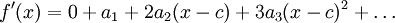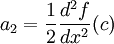# A Motivating Problem

Previous: Taylor Series

Next: The Taylor Series

## A Motivating Problem

In the previous lesson, we found that if a power serieshas a radius of convergence, R, then the infinite series represents a function. But, can we instead ask the opposite question? That is, if we were given a function, f(x), can we find a power series representation for that function? Moreover, what properties does our function have to have in order to have this power series representation? These are questions that we can answer with a short mathematical exploration.

## The Taylor Series

Let's assume we are given a function, f(x), and we will see if there exists a power series representation for that function.

We begin by assuming that we can let f(x) be represented by a power series:But, we do not have the coefficients a0, a1, a2, ... and need to find a way to calculate them.

As a first step, substituting x = c into our power series yields a0 = f(c). To get the next coefficient, a1, assuming we are able to differentiate our given function, we would obtainSubstituting x = c into this expression gives us an expression for a1,So far, we have two of the coefficients, a0 and a1. Continuing with this process, provided that we are able to differentiate our given function again, we would obtain:Again, substituting x = c into this expression yieldsContinuing with this process, we can find as many coefficients as we need, provided that we can differentiate our given function as many times as needed. In other words, if we can differentiate our function N times, we can calculate N power series coefficients, and calculate the series expansion coefficients up to N terms. If we can differentiate our function an infinite number of times, we can find a power series expansion with an infinite number of terms.

The specific power series representation we introduced here derived what is known as the Taylor Series expansion. We will later discuss the conditions that are necessary for a function to have a Taylor series.

## Key Questions for This Lesson

We put these conclusions together into a definition on the next page. But from our simple investigation, we can now ask:

1. are there more efficient ways of calculating the power series expansion of a given function, and
2. does the power series expansion that we find for a given function equal the given function?

You should be able to find answers to both of these questions within this lesson.

Previous: Taylor Series

Next: The Taylor Series